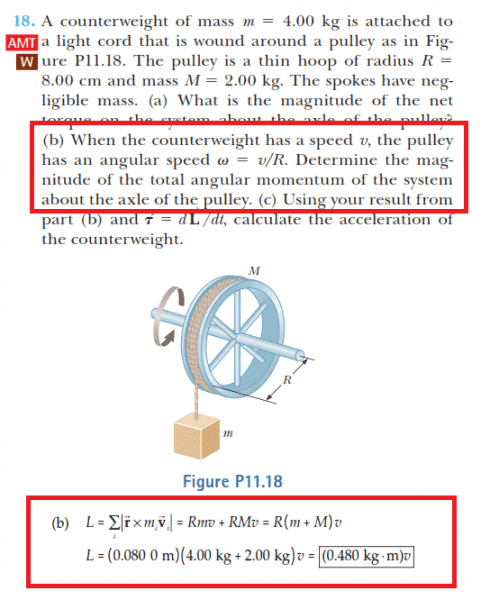# Angular Momentum of a Particle Confusion

• Speedking96
In summary, the angular momentum for a particle can be calculated using the equation L = r x p, while for a rigid body, the equation L = Iw must be used. The pulley is treated as a rigid body, and when calculating its angular velocity, the tangential velocity must be used. This can be obtained by summing the contribution of all particles of the rigid body using the fact that the angular frequency is the same for all of them.

## Homework Statement

I was reading the textbook section on angular momentum, and I'm having some difficulty grasping angular momentum.

Here is a question:In the book, it says that the angular momentum L is equal to vector r cross vector p for a particle. But, for a rigid body, the equation is L = Iw.

In this case, why isn't the pulley treated as a rigid object. Does this mean that you can take the cross product to find the angular momentum in any case?

If someone could please clarify this. Thank you.

#### Attachments

L=r×p ::this equation means rotational
Angular momentum is the. Moment of linear momentum.
L=Iw:: this is analogous to p=MV.but
Thing to be remembered is I can be found for rigid body only. If body is non rigid we have to find L of each particle at any
Instance and add. So this equation is used generally for rigid bodies only. That doesn't imply u can't use the first equation for rigid bodies. only you should be carefull about taking correct value of r .

•Speedking96
Speedking96 said:
In this case, why isn't the pulley treated as a rigid object. Does this mean that you can take the cross product to find the angular momentum in any case?

If someone could please clarify this. Thank you.
The pulley is treated as a rigid body. What is the angular momentum for a ring? How is the angular velocity of the pulley related to the linear velocity of its rim?

For an assembly of particles ##\vec L = \Sigma {\vec r_i \times \vec p_i} = \Sigma {m_i \vec r_i \times \vec v_i}##. For a rigid body rotating with vector ##\vec \omega##, ##\vec v_i= \vec \omega \times \vec r_i ##, so ##\vec L = \Sigma {m_i \vec r_i \times (\vec \omega \times \vec r_i)}##. If ##\vec s_i## is the component of ##\vec r_i## orthogonal to ##\omega##, this reduces to ##\vec L = \Sigma {m_i (\vec s_i^2) \vec \omega} = \vec \omega\Sigma {m_i (\vec s_i^2)} = \vec \omega I##

•Speedking96
ehild said:
The pulley is treated as a rigid body. What is the angular momentum for a ring? How is the angular velocity of the pulley related to the linear velocity of its rim?
When pulley is treated as rigid body and if you know linear velocity if any point ,then divide that velocity with perpendicular distance from axis of rotation and you'll get angular velocity.

ehild said:
Ooops

ehild said:
The pulley is treated as a rigid body. What is the angular momentum for a ring? How is the angular velocity of the pulley related to the linear velocity of its rim?

The angular momentum for a ring is MR2.

Since a ring is a rigid body, it's angular momentum is I = lw = (MR2)(w) = (MR2)(v/r) = mvr

Ok, I see.

So from what I can understand from the posts above, you can use r x p for a particle, or for a sum of particles, but for a rigid body, you must use I = Iw.

And, when the velocity used in the calculations must be the tangential velocity.

Is this correct?

Speedking96 said:
So from what I can understand from the posts above, you can use r x p for a particle, or for a sum of particles, but for a rigid body, you must use I = Iw.

And, when the velocity used in the calculations must be the tangential velocity.

Is this correct?
Yes, L=Iw. And you get it by summing (integrating) the contribution rxp of all particles of that rigid body, using the fact that the angular frequency is the same for all of them.

•Speedking96
Thank you.